# 概论

1. 定义损失函数。
2. 使用优化算法最小化损失。优化过程中，损失函数就是优化算法的目标函数

# 优化的目标

1. 优化的目标是减少训练误差，最小化目标函数是基于训练集的损失函数。
2. 深度学习的目标是减少泛化误差，是在有限数据集上寻找到合适的模型。

g

g

g和实际风险函数

f

f

f，在训练数据集中最低的经验风险可能与最低的真实风险（泛化误差）是不同的。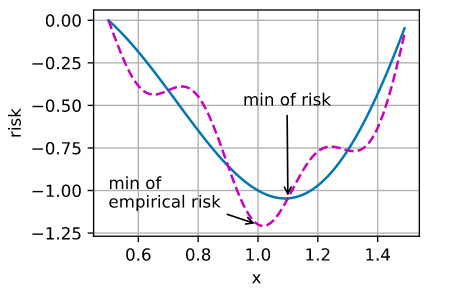## 优化的挑战

### 局部最小值

f

(

x

)

f(x)

f(x)，如果在

x

x

x处对应的

f

(

x

)

f(x)

f(x)值小于在

x

x

x附近任何其他点的

f

(

x

)

f(x)

f(x)值，那么

f

(

x

)

f(x)

f(x)可能是局部最小值。如果

f

(

x

)

f(x)

f(x)

x

x

x处的值是整个域上目标函数的最小值，那么

f

(

x

)

f(x)

f(x)是全局最小值。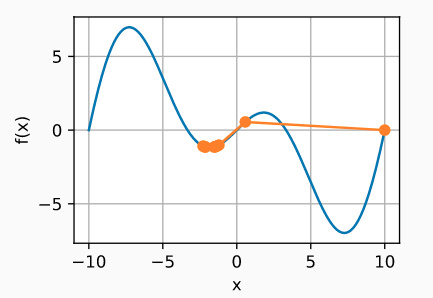## 鞍点

f

(

x

)

=

x

3

f(x) = x^3

f(x)=x3。它的一阶和二阶导数在

x

=

0

x=0

x=0时消失。这时优化可能会停止，尽管它不是最小值。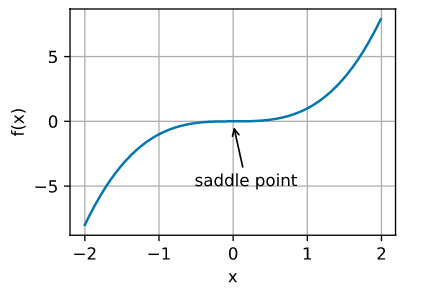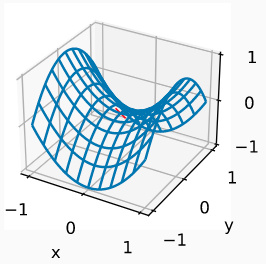k

k

k维向量，其输出是标量，因此其Hessian矩阵（也称黑塞矩阵）将有

k

k

k特征值（参考online appendix on eigendecompositions)。函数的解决方案可以是局部最小值、局部最大值或函数梯度为零的位置处的鞍点：

• 当函数在零梯度位置处的Hessian矩阵的特征值全部为正值时，我们有该函数的局部最小值。
• 当函数在零梯度位置处的Hessian矩阵的特征值全部为负值时，我们有该函数的局部最大值。
• 当函数在零梯度位置处的Hessian矩阵的特征值为负值和正值时，我们对函数有一个鞍点。

### 梯度消失

f

(

x

)

=

tanh

(

x

)

f(x) = \tanh(x)

f(x)=tanh(x)，然后我们恰好从

x

=

4

x = 4

x=4开始。正如我们所看到的那样，

f

f

f的梯度接近零。更具体地说，

f

(

x

)

=

1

tanh

2

(

x

)

f'(x) = 1 - \tanh^2(x)

f(x)=1tanh2(x)，因此是

f

(

4

)

=

0.0013

f'(4) = 0.0013

f(4)=0.0013。因此，在我们取得进展之前，优化将会停滞很长一段时间。事实证明，这是在引入ReLU激活函数之前训练深度学习模型相当棘手的原因之一。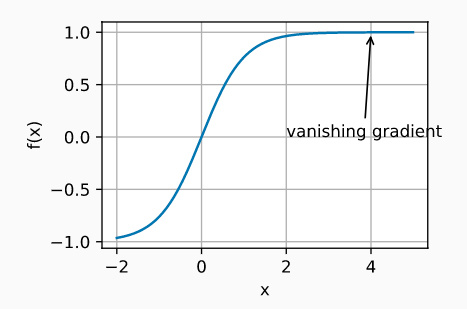# 总结

THE END